Integers 2
(10 Questions)
CA Math Standards
Grade 5
2.1 Add, subtract, multiply and divide with decimals; add with negative numbers; subtract positive integers from negative integers; verify the reasonableness of the results.
From Mr. Anker Tests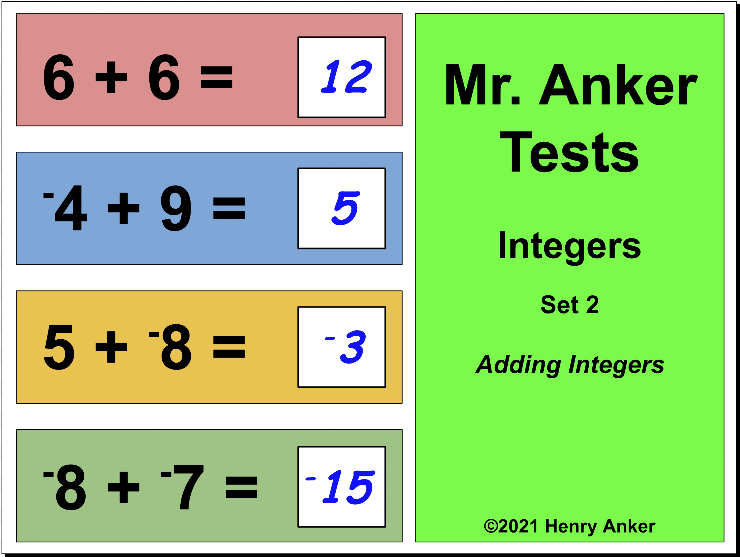Integer Number Line Reference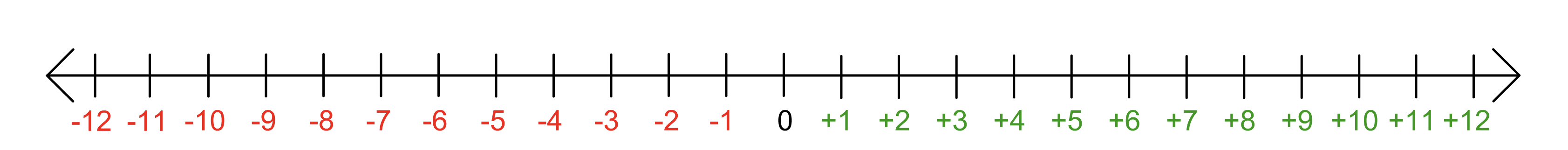*Note
Positive (+) signs will no longer appear next to positive integers. Only negative integers will have a negative sign (-) next to the number.
1. Which of the choices below is the correct solution to this equation? *
1 point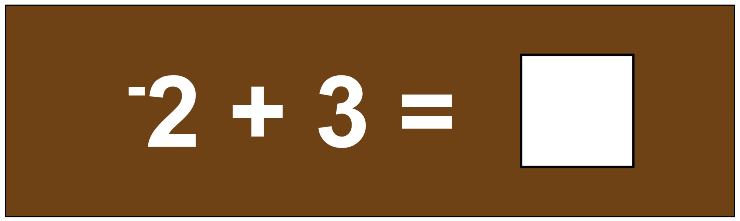2. In the space provided below, type the correct solution to this equation. *
1 point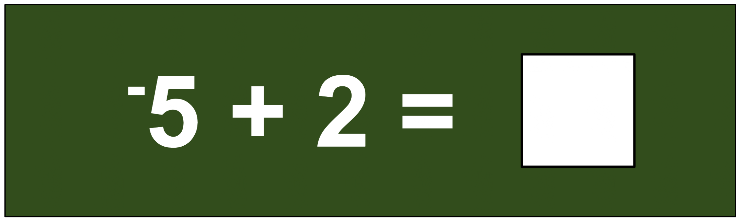3. Which of the choices below is the correct solution to this equation? *
1 point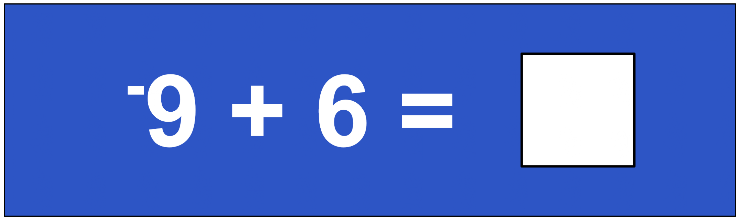4. In the space provided below, type the correct solution to this equation. *
1 point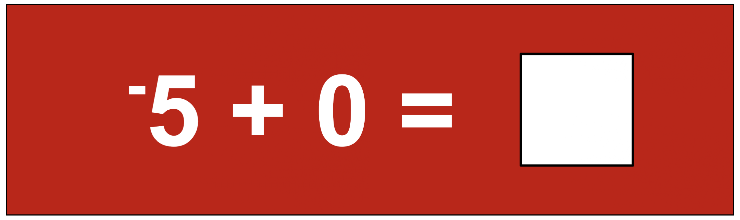5. Which of the choices below is the correct solution to this equation? *
1 point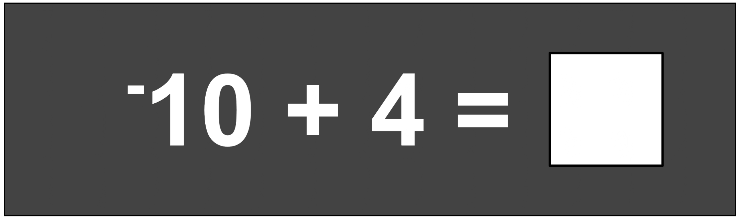6. In the space provided below, type the correct solution to this equation. *
1 point7. Which of the choices below is the correct solution to this equation? *
1 point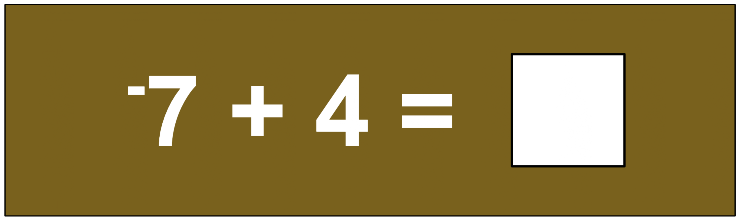8. In the space provided below, type the correct solution to this equation. *
1 point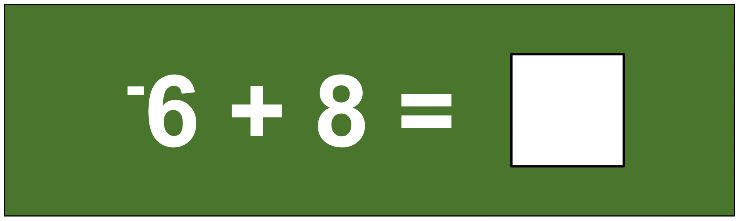9. Which choice makes the number sentence true? *
1 point10. In the space provided below, type the correct solution to this equation. *
1 point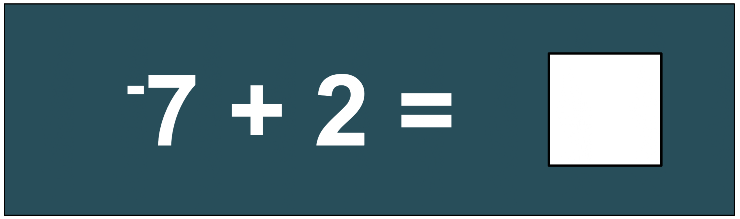Never submit passwords through Google Forms.
This content is neither created nor endorsed by Google. Report Abuse - Terms of Service - Privacy Policy